Question

# A coin with probability p of heads is tossed until the first head occurs. It is...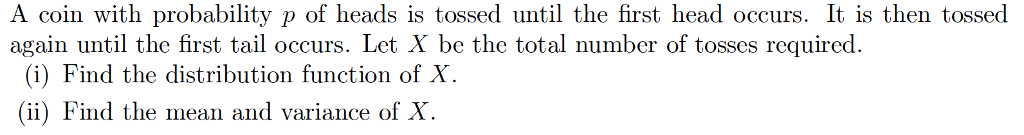A coin with probability p of heads is tossed until the first head occurs. It is then tossed again until the first tail occurs. Let X be the total number of tosses required. (i) Find the distribution function of X. (ii) Find the mean and variance of X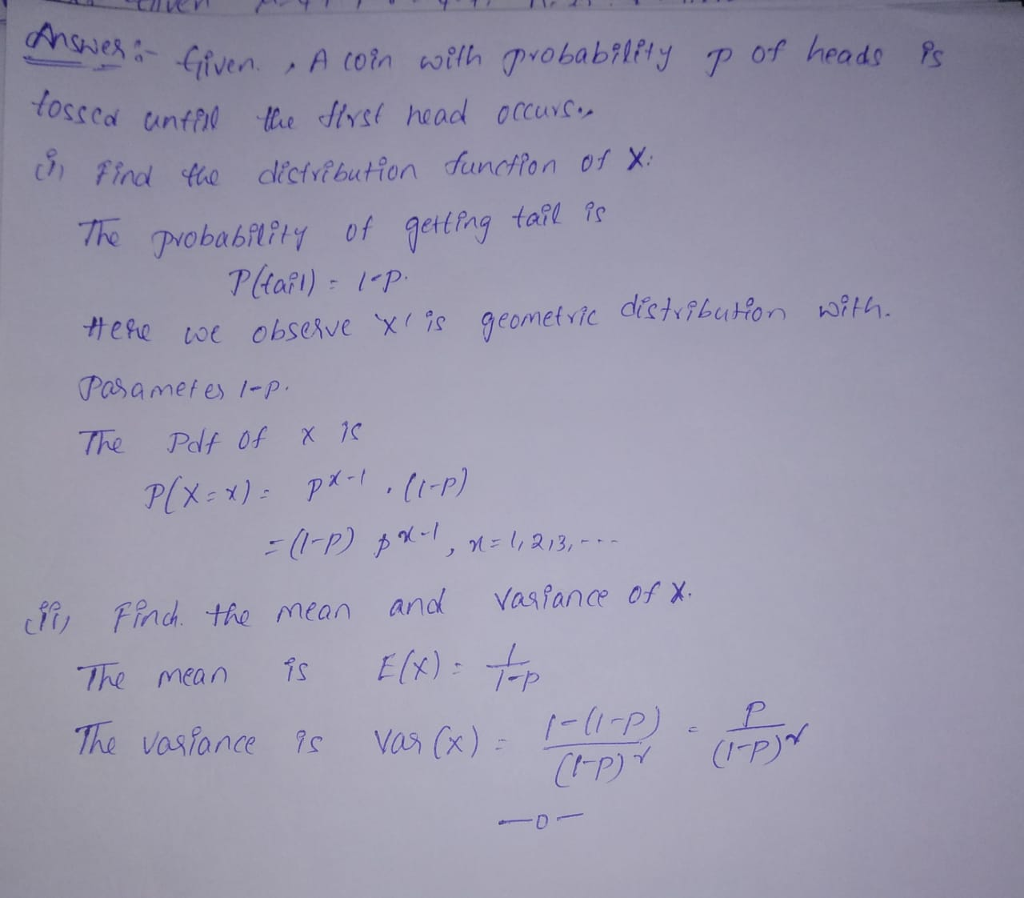#### Earn Coins

Coins can be redeemed for fabulous gifts.

Similar Homework Help Questions
• ### A coin with probability p is tossed until the first head occurs. It is then tossed...

A coin with probability p is tossed until the first head occurs. It is then tossed again until the first tail occurs. Let X be the total number of tosses required. 1) Find the distribution function of X. 2) Find the mean and variance of X.

• ### E. A coin with probabiltiy p of heads is tossed till the first head occurs. It...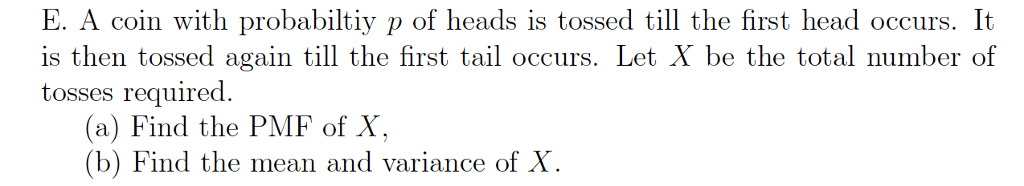E. A coin with probabiltiy p of heads is tossed till the first head occurs. It 1S is then tossed again till the first tail occurs. Let X be the total number of tosses required (a) Find the PMF of X, (b) Find the mean and variance of X

• ### ===============================================================================================================================================================================================================================================================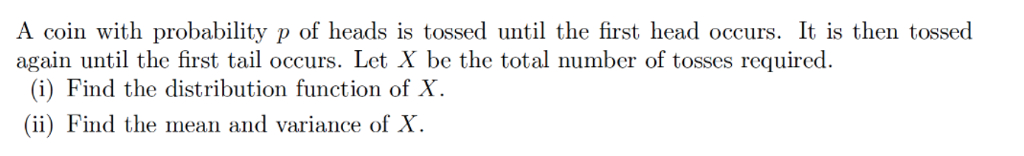======================================================================================================================================================================================================================================================================================= There are two steps in the description of this problem. First, toss the coin until a head appears. Then, toss the coin until a tail appears. It is NOT "toss a coin until a head appears" problem. ======================================================================================================================================================================================================================================================================================= A coin with probability p of heads is tossed until the first head occurs. It is then tossed again until the first tail occurs. Let X be the total number of tosses required (i) Find the distribution function of X (ii)...

• ### A biased coin is tossed until a head occurs. If the probability of heads on any...

A biased coin is tossed until a head occurs. If the probability of heads on any given toss is .4, What is the probability that it will take 7 tosses until the first head occurs? The answer i got was , (.60)^2(.40) Now for the second part it says, what is the probability that it will take 9 tosses until the second head occurs. Is the answer for this part be 9C2(.40)^2(.60)^7 or 8C1(.40)(.60)^5 I can't figure out if its...

• ### If the coin is unbalanced and a head has a 30% chance of occurring, find the joint probability distribution f(w, z)

A coin is tossed twice. Let Z denote the number of heads on the first toss and let W denote the total number of heads on the two tosses. If the coin is unbalanced and a head has a 30% chance of occurring, find the joint probability distribution f(w, z)

• ### A fair coin is tossed 20 times. Let X be the number of heads thrown in...

A fair coin is tossed 20 times. Let X be the number of heads thrown in the first 10 tosses, and let Y be the number of heads tossed in the last 10 tosses. Find the conditional probability that X = 6, given that X + Y = 10.

• ### A fair coin is tossed 20 times. Let X be the number of heads thrown in...

A fair coin is tossed 20 times. Let X be the number of heads thrown in the first 10 tosses, and let Y be the number of heads tossed in the last 10 tosses. Find the conditional probability that X = 6, given that X + Y = 10.

• ### A fair coin is flipped independently until the first Heads is observed. Let the random variable...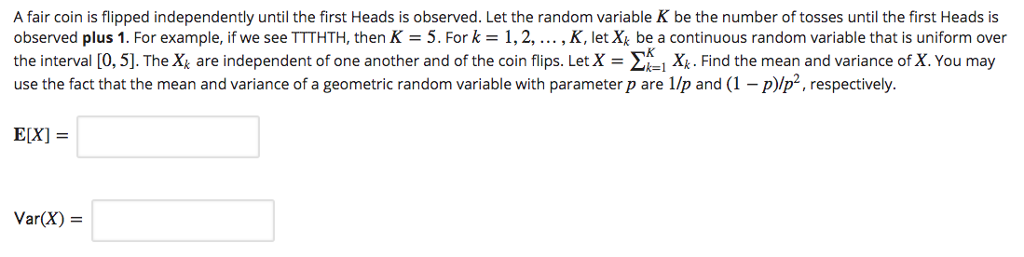A fair coin is flipped independently until the first Heads is observed. Let the random variable K be the number of tosses until the first Heads is observed plus 1. For example, if we see TTTHTH, then K = 5. For k 1, 2, , K, let Xk be a continuous random variable that is uniform over the interval [0, 5]. The Xk are independent of one another and of the coin flips. LetX = Σ i Xo Find the...

• ### h-0 h-0 h=0 or otherwise. (Oxford 1982M) 9. The probability of obtaining a head when a...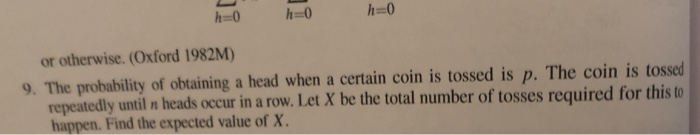h-0 h-0 h=0 or otherwise. (Oxford 1982M) 9. The probability of obtaining a head when a certain coin is tossed is p. The coin is tossed repeatedly until n heads occur in a row. Let X be the total number of tosses required for this to happen. Find the expected value of X.

• ### A coin will be tossed multiple times. Probability of head is 1/2, and probability of tail is 1/2....

A coin will be tossed multiple times. Probability of head is 1/2, and probability of tail is 1/2. they are independent from each other. X is a random variable that counts how often the coin must be tossed until the first head appears. calculate for all k=1,2,3,..., how big the probability is for: i) X=k ii) X>k iii) X<k